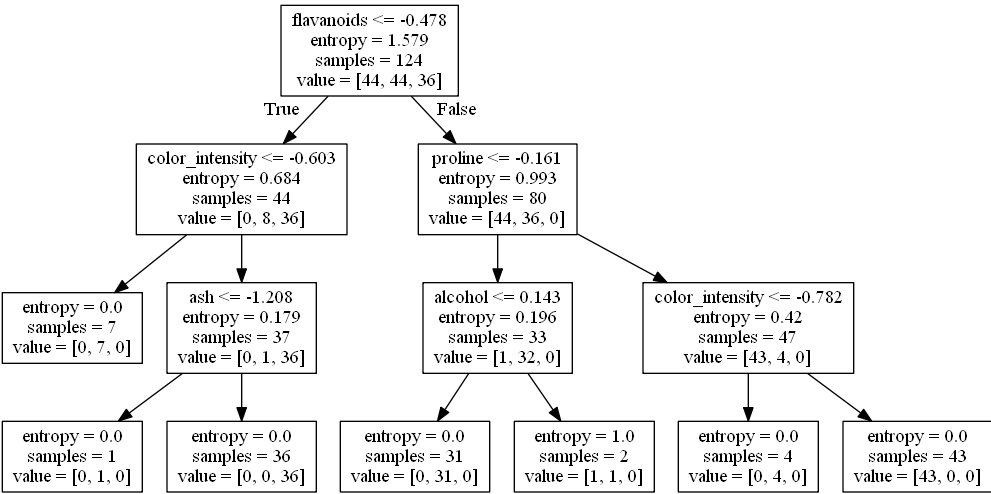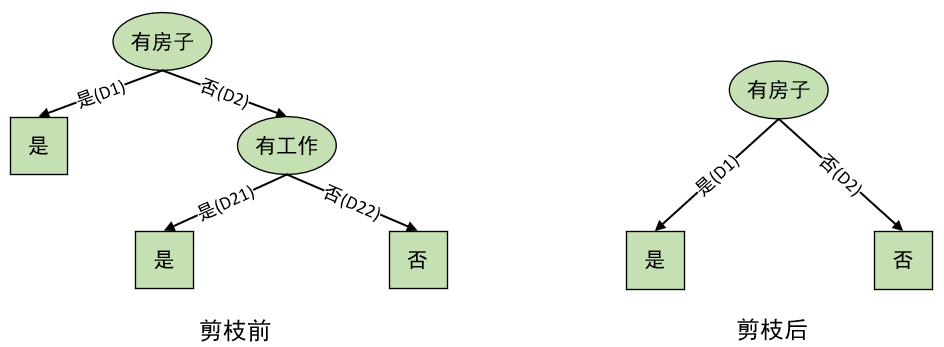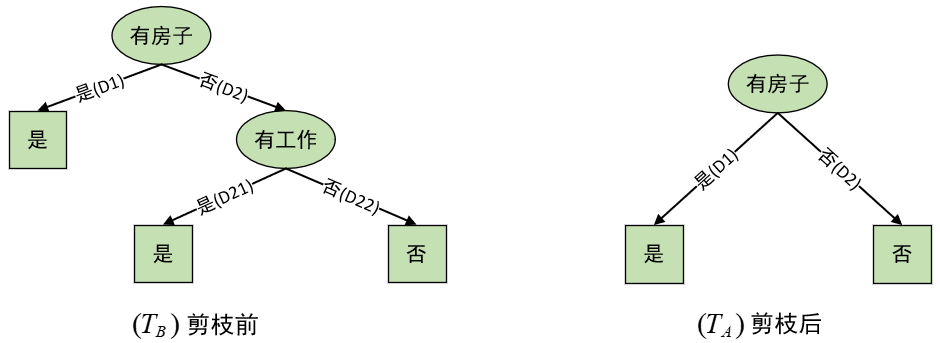# 决策树的建模与剪枝

## 1 Scikit-learn建模

### 1.1 Scikit-learn接口介绍

• 第一步：建立模型

这一步通常来说都是通过在对应的路径下导入我们需要用到的模型类，例如:

from sklearn.linear_model import SGDClassifier

在导入模型类后，我们需要通过这个类来实例化一个模型对应的类对象并填入对应超参数，例如：

model = SGDClassifier(loss='log', penalty='l2', alpha=0.5)

• 第二步：训练模型

在sklearn中，所有模型的训练（或者计算）过程都是通过.fit()这个方法来完成的。并且一般情况下需要按实际情况在调用.fit()时传入相应参数。如果是有监督模型则一般是model.fit(x,y)，无监督则是mode.fit(x)。同时，还可可以调用model.score(x,y)来对模型的结果进行评估。

• 第三步：模型预测

在训练好一个模型后，通常都要对测试集或者新输入的数据进行预测。在sklearn中一般都是通过模型类对应的.predict(x)方法来实现的。

### 1.2 决策树建模

• 导入数据集

本次示例用到的数据集为sklearn中内置的一个数据集wine，这是一个比较常见的数据集，包含3个类别、178个样本和13个特征维度。

from sklearn.datasets import load_wine
x, y = data.data, data.target
x_train, x_test, y_train, y_test = \
train_test_split(x, y, test_size=0.3,
shuffle=True, random_state=20)
if scale:
ss = StandardScaler()
x_train = ss.fit_transform(x_train)
x_test = ss.transform(x_test)
return x_train, x_test, y_train, y_test

• 定义模型

再导入数据后就可以进行建模训练。

from sklearn.tree import DecisionTreeClassifier
def train(x_train, x_test, y_train, y_test):
model = DecisionTreeClassifier(criterion="entropy")
model.fit(x_train, y_train)
model.predict()
print("Accuracy: ", model.score(x_test, y_test))

if __name__ == '__main__':
x_train, x_test, y_train, y_test = load_data()
train(x_train, x_test, y_train, y_test)
#输出结果
Accuracy:  0.9629629629629629


在上面的第3行代码中，参数criterion='entropy'表示使用信息增益作为决策树每个划分时的标准。遗憾的是在sklearn中并没有实现以信息增益作为划分标准时的情况。

### 1.3 决策树可视化

from sklearn.tree import DecisionTreeClassifier,export_graphviz
def train(x_train, x_test, y_train, y_test):
model = DecisionTreeClassifier(criterion="entropy",max_depth=3)
model.fit(x_train, y_train)
with open("visualization.dot", "w") as f:
f = export_graphviz(model, feature_names=feature_names, out_file=f)
print("Accuracy: ", model.score(x_test, y_test))## 2 决策树剪枝

### 2.1 剪枝思想### 2.2 剪枝

C α ( T ) = ∑ t = 1 ∣ T ∣ N t H t ( T ) + α ∣ T ∣ (1) C_{\alpha}(T)=\sum_{t=1}^{|T|}N_tH_t(T)+\alpha|T|\tag{1}

H t ( T ) = − ∑ k N t k N t log ⁡ N t k N t (2) H_t(T)=-\sum_k\frac{N_{tk}}{N_t}\log{\frac{N_{tk}}{N_t}}\tag{2}

C ( T ) = ∑ t = 1 ∣ T ∣ N t H t ( T ) = − ∑ t = 1 ∣ T ∣ ∑ k = 1 K N t k log ⁡ N t k N t (3) C(T)=\sum_{t=1}^{|T|}N_tH_t(T)=-\sum_{t=1}^{|T|}\sum_{k=1}^KN_{tk}\log{\frac{N_{tk}}{N_t}}\tag{3}

C α ( T ) = C ( T ) + α ∣ T ∣ (4) C_{\alpha}(T)=C(T)+\alpha|T|\tag{4}

（1）计算每个叶结点的经验（信息）熵；

（2）递归地从树的叶结点往上回溯；设一组叶结点回溯到其父结点之前与之后的整体树分别为 T B , T A T_B,T_A ，其对应的损失函数值分别是 C α ( T B ) , C α ( T A ) C_{\alpha}(T_B),C_{\alpha}(T_A) ，如果 C α ( T A ) ≤ C α ( T B ) C_{\alpha}(T_A)\leq C_{\alpha}(T_B) 则进行剪枝，即将父结点变为新的叶结点。

（3）返回（2），直到不能继续为止，得到损失函数最小的子树 T α T_{\alpha}I D 年龄 有工作 有自己的房子 贷款情况 类别 3 青年 是 否 好 是 13 老年 是 否 好 是 D 21 14 老年 是 否 非常好 是 1 青年 否 否 一般 否 2 青年 否 否 好 否 5 青年 否 否 一般 否 D 22 6 中年 否 否 一般 否 7 中年 否 否 好 否 15 老年 否 否 一般 否 \begin{array}{c|cc}\hline ID&\text{年龄}&\text{有工作}&\text{有自己的房子}&\text{贷款情况}&\text{类别}\\\hline3&\text{青年}&\text{是}&\text{否}&\text{好}&\text{是}\\13&\text{老年}&\text{是}&\text{否}&\text{好}&\text{是}&D_{21}\\14&\text{老年}&\text{是}&\text{否}&\text{非常好}&\text{是}\\\hline1&\text{青年}&\text{否}&\text{否}&\text{一般}&\text{否}\\2&\text{青年}&\text{否}&\text{否}&\text{好}&\text{否}\\5&\text{青年}&\text{否}&\text{否}&\text{一般}&\text{否}&D_{22}\\6&\text{中年}&\text{否}&\text{否}&\text{一般}&\text{否}\\7&\text{中年}&\text{否}&\text{否}&\text{好}&\text{否}\\15&\text{老年}&\text{否}&\text{否}&\text{一般}&\text{否}\\\hline\end{array}

C α ( T B ) = C ( T B ) + α ∣ T B ∣ C ( T B ) = − ∑ t = 1 2 ∑ k = 1 2 N t k log ⁡ N t k N t = − [ ( 3 log ⁡ 3 3 + 0 ) + ( 6 log ⁡ 6 6 + 0 ) ] = 0 C α ( T B ) = 0 + α × 2 C α ( T A ) = C ( T A ) + α ∣ T A ∣ C ( T A ) = − ∑ t = 1 1 ∑ k = 1 2 N t k log ⁡ N t k N t = − [ ( 3 log ⁡ 3 9 + 6 log ⁡ 6 9 ) ] ≈ 8 C α ( T A ) = 8 + α × 1 \begin{aligned} &C_{\alpha}(T_B)=C(T_B)+\alpha|T_B|\\[1ex] &C(T_B)=-\sum_{t=1}^{2}\sum_{k=1}^2N_{tk}\log{\frac{N_{tk}}{N_t}}=-\left[(3\log{\frac{3}{3}}+0)+(6\log{\frac{6}{6}}+0)\right]=0\\[1ex] &C_{\alpha}(T_B)=0+\alpha\times 2\\[3ex] &C_{\alpha}(T_A)=C(T_A)+\alpha|T_A|\\[1ex] &C(T_A)=-\sum_{t=1}^{1}\sum_{k=1}^2N_{tk}\log{\frac{N_{tk}}{N_t}}=-\left[(3\log{\frac{3}{9}}+6\log{\frac{6}{9}})\right]\approx 8\\[1ex] &C_{\alpha}(T_A)=8+\alpha\times1 \end{aligned}

## 3 总结

### 引用

《统计机器学习（第二版）》李航，公众号回复“统计学习方法”即可获得电子版与讲义

《Python与机器学习实战》何宇健

 示例代码 ： https://github.com/moon-hotel/MachineLearningWithMe07-06180
06-13192108-091万+
12-061万+
05-043895
04-054250
08-253万+
05-2090
11-033872
08-171万+
11-194802
12-302万+
02-252万+
08-112万+
07-204962